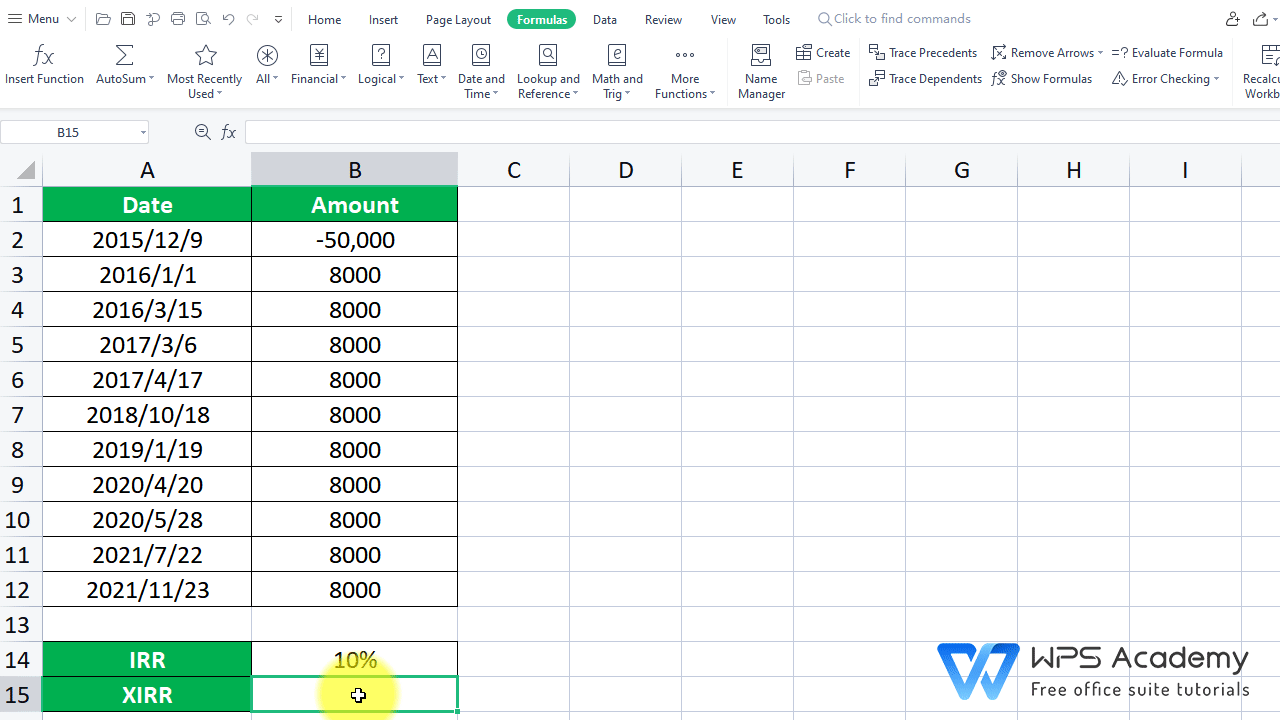XIRR Function in EXCEL

January 5, 2022
85 Views

Graphic skills

· Description:

IRR means the Internal Rate of Return that is represented by numbers for a series of cash flows. Compared with the IRR function, the XIRR function can calculate the IRR of irregular intervals, which can return the internal rate of return for a schedule of cash flows.

· Syntax:

(Value, dates, guess)

· Arguments:

Value is a series of cash flows that correspond to a schedule of payments in dates.

Dates is a schedule of payment dates that corresponds to the cash flow payments.

Guess is a number that you guess is close to the result of IRR; 0.1 (10 percent) of omitted.

· Example:

Suppose we want to calculate the IRR of this investment project with an initial investment of 50,000 and a yearly income of 8000. This is similar to our previous case in the IRR function tutorial. The difference is the cash flows occur at irregular intervals.

2. Select cell B15 where we want to insert the function. Then head to the Formulas tab > Insert Function > enter XIRR in the edit box, or just type “=XIRR” in the cell.

3. Here we want to insert the XIRR function.1) Value is a series of cash flows that correspond to a schedule of payments in dates. Column B2:B12 is the area that contains the cash flow amounts. So let's put B2:B12 at Value.

2) Dates is a schedule of payment dates that corresponds to the cash flow payments. Column A2:A12 is the area that contains the dates that correspond to Value. So let's put A2:A12 at Dates.

3) Guess is a number that you guess is close to the result of IRR; 0.1 (10 percent) of omitted. The third argument is a guessed number that is close to the expected internal rate of return, which we choose to omit.

In the pop-up dialog, enter B2:B12 at Value, A2:A12 at Date. Then click OK.

We can get 20.17% as the result, which is the IRR of this investment project would give after 10 years. The difference of cell B14 and B15 indicates that the IRR function fails to calculate when dates are irregularly listed.